You are here: Glossary - Historical Reports

Glossary - Historical Reports

The following tables provide metrics definitions for all the metrics available in the agent and queue interactions reports:

Agent Interactions - Summary

Report Category Report Template Metrics Metrics Definitions Formula
Agent Interactions Summary Presented The total number of interactions offered to the agent (includes interactions that were stil presenting in the next interval)

Dimensions:

• Time
• Agent
• Media
• Channel
• Queue
Accepted The total number of interactions accepted by the agent
Rejected The total number of interactions rejected by the agent
Abandoned The total number of interactions abandoned by a customer while being presented to the agent
Reject Timeout the total interactions presented to the agent, but for which the agent doesn't take action during the allocated presentation time
Offering The total number of interactions that are currently being presented to the agent (when an interval has ended but an interaction is stil being presented to the agent)
Accepted % The percentage of interactions answered by the agent over the total number of interactions that entered the queue Accepted/Entered*100
Rejected % The total number of interactions rejected by the agent Rejected/Entered*100
Abandoned % The percentage of interactions abandoned by the agent over the total number of interactions that entered the queue Abandoned/Entered*100
Offering Time The total time an interaction has spent being offered to the agent (from the moment the interaction is presented to the agent until the agent takes an action or the system stops presenting the interaction to the agent (such as if the presentation time expires))
Handling Time The total time the agent has spent in the Handling state (from the time that an interaction is accepted by an agent until it is terminated)
Wrap Up Time The total time the agent has spent in the Wrap Up state post-processing an interaction (from the time that an accepted interaction is terminated until it is wrapped up)
Busy Time The total time the agent has spent in the Offering, Handling, and Wrap Up states
Avg Offering Time The average time spent by an agent while being offered an interaction (also called Avg Speed to Answer)
Average Handling Time The average time spent by an agent in the Handling state (includes hold time)
Average Wrap Up Time The average time spent by an agent in the Wrap Up state
Average Busy Time The average time the agent has spent in the Offering, Handling, and Wrap Up states
Longest Offering Time The longest time it takes to accept an interaction (compared to all the interactions that entered a queue) from the time it is offered to the time it is accepted or rejected by an agent

Agent Interactions - Call Summary

Report Category Report Template Metrics   Formula
Agent Interactions Call Summary direct inbound The total number of direct calls to the agent, excluding agent-to-agent calls

Dimensions:

• Time
• Group
• Agent
• Media
• Channel
• Queue
direct inbound time The total time (includes Offering + Handling + Wrap Up) spent on direct calls to the agent, excluding agent-to-agent calls (this is the equivalent of busy time for inbound queues, but for agent direct inbound calls)
avg direct inbound time The average time spent by an agent on direct calls, excluding agent-to-agent calls
direct outbound The total number of calls made by the agent, excluding outbound queue calls and agent-to-agent calls
direct outbound time The total time (includes Offering + Handling + Wrap Up) spent on calls made by the agent, excluding outbound queue calls and agent-to-agent calls (the equivalent of busy time for outbound queues, but for agent direct outbound calls)
avg direct outbound time The average time spent by an agent on outbound calls made by an agent, excluding outbound queue calls and agent-to-agent calls
internal calls The total number of agent-to-agent calls initiated or received
internal calls time The total time (includes Offering + Handling + Wrap Up) spent on agent-to-agent calls
Internal calls initiated The total time (includes Offering + Handling + Wrap Up) spent on agent-to-agent calls, but only for calls initiated by the agent
Internal calls received The total time (includes Offering + Handling + Wrap Up) spent on agent-to-agent calls, but only for calls received by the agent
Hold The total number of call holds the agent has performed
Hold time The total time the agent has spent placing a customer or agent on hold on any line
Longest Hold Time The longest time the agent has spent placing a customer or agent on hold on any line
Consultations established The number of times the agent successfully established an outbound call, while another call is on hold, by an agent (this counter does not decrease the "outbound direct interaction established" value, which increases at the same time as this counter)
Conferences The total number of conferences (join lines) the agent was part of
Conference time The total time spent on conference calls by the agent (the time since the conference has been established to the time the interactions ends (one of the last 2 participants hangs up))
Conferences established The total number of conferences (join lines) the agent has initiated
Conferences established time The total time spent by the agent in conference calls that the agent has initiated
Conferences received The total number of conferences (join lines) the agent has received
Conferences received time The total time spent by the agent in conference calls that the agent has received
Transfers initiated The total number of transfers initiated by an agent (either to an agent or to a queue via blind/warm transfer)
Transfers received The total number of transfers received by an agent (either to an agent or to a queue via blind/warm transfer)
Blind Transfers Initiated The total number of blind transfers initiated by an agent (to an agent or to a queue)
Blind Transfer to Queue The total number of blind transfers to queues initiated by an agent
Blind Transfer to Agent The total number of blind transfers initiated and received by an agent (outside of a queue)
Blind Transfers Received The total number of blind transfers received by an agent
Warm Transfers Completed (warm transfer initiated) The total number of warm transfers initiated by an agent
Warm Transfers Received The total number of warm transfers received by an agent

Agent Interactions - Handling and Wrap Up

Report Category Report Template Metrics Metrics Definitions Formula
Agent Interactions Handling and Wrap up Offering Time The total time the agent has spent in the Offering state (from the time that an interaction is presented to the agent until it is accepted)

Dimensions:

• Time
• Agent
• Media
• Channel
• Queue
Avg Offering Time The average time the agent has spent in the Offiering state (also called AVG SPEED OF ANSWER)
Handling Time The total time the agent has spent in the Handling state (from the time that an interaction is accepted by an agent until it is terminated)
Avg Handling Time The average time the agent has spent in the Handling state (includes hold time)
Wrap Up Time The total time the agent has spent in the Wrap Up state (from the time that an accepted interaction is terminated until it has been wrapped up)
Avg Wrap Up Time The average time the agent has spent in the Wrap Up state
Processing Time The total time the agent has spent in the Handling and Wrap Up states
Avg Processing Time The average time the agent has spent in the Handling and Wrap Up states
Busy Time The total time the agent has spent in the Offering, Handling, and Wrap Up states
Avg Busy Time The average time the agent has spent in the Handling, Wrap Up, and Offering states

Agent Interactions - By Wrap up Codes

Report Category Report Template Metrics Metrics Definitions Formula
Agent Interactions By Wrap up Codes Count The total number of times the transaction code has been used

Dimensions:

• Time
• Agent
• Media
• Channel
• Queue
Time The total time spent processing (Handling and Wrap Up) interactions by an agent that finished with them using the transaction code

Agent Status - Time on Status

Report Category Report Template Metrics Metrics Definitions Formula
Agent Status Time on Status Available Time The total time the agent has spent in the Available state

Dimensions:

• Time
• Agent
• Media
• Channel
• Queue
Available Time % The percentage of time the agent has spent in the Available state over the Logged In Time
Handling Time The total time the agent has spent in the Offering and Handling states (from the moment the interaction is accepted by the agent until it is terminated)
Handling Time % The percentage of time the agent has spent in the Offering and Handling states over the Logged In Time
Wrap Up Time The total time the agent has spent in the Wrap Up state (from the moment the accepted interaction is terminated until it is wrapped up)
Wrap Up Time % The percentage of time the agent has spent in the Wrap Up state over the Logged In Time
Working Offline Time The total time the agent has spent in the Work Offline state
Working Offline Time % The percentage of time the agent has spent in the Work Offline state over the Logged In Time
On Break Time The total time the agent has spent in the On Break state
On Break Time % The percentage of time the agent has spent in the On Break state over the Logged In Time
Busy Time The total time the agent has spent in the Handling and Wrap Up states
Busy Time % The percentage of the time the agent has spent in the Handling and Wrap Up states over the Logged In Time
Logged In Time Time The total time the agent has spent in the Logged In state
By Status Code Time The total time the agent has spent in the selected state

Queue Interactions - Summary

Report Category Report Template Metrics Metrics Definitions Formula
Queue Interactions Summary Entered The total number of interactions that entered the queue

Dimensions:

• Time
• Agent
• Media
• Channel
• Queue
Accepted The total number of interactions answered by the agent
Accepted in SLA The total number of interactions answered by all agents under the SLA Threshold Time
Accepted in SLA % The percentage of interactions answered by all agents under the SLA Threshold Time over the number of all accepted interactions
SLA % The actual SLA% defined as a percentage of "number of interactions accepted by agents before the SLA waiting time threshold is reached" divided by the "number of interactions that entered the queue" multiplied by 100.
Average waiting time for accepted transactions The average time the interaction has spent in the queue waiting to be served (time starts from the point the interaction enters the queue to when it is offered to the agent)
Longest Wait in queue The time of the current longest wait to be served in the queue Accepted/Entered*100
Average Wait in queue The average time the interaction has spent in the queue waiting to be served (time starts from the point the interaction enters the queue to when it is offered to the agent)
Handling Time The time the agent has spent in the Handling state (from the time that an interaction is accepted by an agent until it is terminated)
Avg Handling Time The average time the agent has spent in the Handling state (includes hold time)
Wrap Up Time The total time the agent has spent in the Wrap Up state
Avg Wrap Up Time The average time the agent has spent in the Wrap Up state
Processing Time The total time the agent has spent in the Handling and Wrap Up states (from the time that an interaction is accepted by an agent until it is wrapped up)
Avg Processing Time The average time the agent has spent in the Handling and Wrap Up states
Busy Time The total time the agent has spent in the Offering, Handling, and Wrap Up states (from the time that an interaction is presented to the agent until it is wrapped up)
Avg Busy Time The average time the agent has spent in the Offering, Handling, and Wrap Up states

Queue Interactions - Abandoned

Report Category Report Template Metrics Metrics Definitions Formula
Queue Interactions Abandoned Entered The total number of interactions that entered the queue

Dimensions:

• Time
• Agent
• Media
• Channel
• Queue
Accepted The total number of interactions answered by the agent
Accepted % The percentage of all interactions answered by the agent over the number of entered interactions
Diverted The total number of interactions that entered and left the queue without being ended (the interactions were forwarded to another queue, transferred, or forwarded within an IVR script)
Diverted % The percentage of all interactions that entered and left the queue without being ended (the interactions were forwarded to another queue, transferred, or forwarded within an IVR script) over the total number of interactions that entered the queue Diverted / Entered * 100
Offering The total number of interactions that are currently being presented to the agent
Offering % The percentage of all currently-presented interactions over the total number of interactions that entered the queue Offering / Entered * 100
Short Abandoned (Inbound and Chat only) All interactions that finish (end) in abandonment but are under the short abandonment threshold Short Abandonment Threshold is set to 5s by default
Short Abandoned % The percentage of all interaction that entered the queue and ended up as being hung up by the customer in under the short abandonment threshold over the total of interactions that entered the queue Short Abandoned / Entered * 100
Abandoned The number of interactions that terminated in the queue and ended up as being hung up by the customer (Excludes short abandoned)
Abandoned % The percentage of interactions that terminated in the queue without being served over the total number of interactions that entered the queue (excludes short abandoned) Abandoned / Entered * 100
Total Abandoned The total number of all interactions that entered the queue and ended up as being hung up by the customer (includes short abandoned) Short Abandoned + Abandoned
Total Abandoned % The percentage of all interactions that ended up as abandonments over the total number of interactions that entered the queue (includes short abandoned) Total Abandoned / Entered * 100
Average Abandon time The average time spent by all interactions in the queue waiting to be served that ended up as an abandonement (includes short abandoned) Average Abandoned Time = (Short Abandon time + Abandon time) / Total Abandoned
Longest Abandon time The longest time an interaction spent waiting in a queue to be served and ended up as an abandonement
< 5s The total number of abandoned interactions under 5 seconds in length
< 5s % The percentage of the Total Abandoned interactions under 5 seconds in length over the total Entered interactions Total Abandoned < 5s / Entered * 100
5s ‒ 10s The total number of abandoned interactions between 5 and 10 seconds in length
5s ‒ 10s % The percentage of the Total Abandoned interactions between 5 and 10 seconds in length over the total Entered interactions Total Abandoned between 5s - 10s / Entered * 100
10s ‒ 20s The total number of abandoned interactions between 10 and 20 seconds in length
10s ‒ 20s % The percentage of the Total Abandoned interactions between 10 and 20 seconds in length over the total Entered interactions Total Abandoned between 10s - 20s / Entered * 100
20s ‒ 30s The total number of abandoned interactions between 20 and 30 seconds in length
20s ‒ 30s % The percentage of the Total Abandoned interactions between 20 and 30 seconds in length over the total Entered interactions Total Abandoned between 20s - 30s / Entered * 100
30s ‒ 45s The total number of abandoned interactions between 30 and 45 seconds in length
30s ‒ 45s % The percentage of the Total Abandoned interactions between 30 and 45 seconds in length over the total Entered interactions Total Abandoned between 20s - 30s / Entered * 100
45s ‒ 1m The total number of abandoned interactions between 45 seconds and 1 minute in length
45s ‒ 1m % The percentage of the Total Abandoned interactions between 45 seconds and 1 minute in length over the total Entered interactions Total Abandoned between 45s - 1m / Entered * 100
1m ‒ 2m The total number of abandoned interactions between 1 and 2 minutes in length
1m ‒ 2m % The percentage of the Total Abandoned interactions between 1 and 2 minutes in length over the total Entered interactions Total Abandoned between 1m - 2m / Entered * 100
2m ‒ 5m The total number of abandoned interactions between 2 and 5 minutes in length
2m ‒ 5m % The percentage of the Total Abandoned interactions between 2 and 5 minutes in length over the total Entered interactions Total Abandoned between 2m - 5m / Entered * 100
5m ‒ 10m The total number of abandoned interactions between 5 and 10 minutes in length
5m ‒ 10m % The percentage of the Total Abandoned interactions between 5 and 10 minutes in length over the total Entered interactions Total Abandoned between 5m - 10m / Entered * 100
> 10m The total number of abandoned interactions over 10 minutes in length
> 10m % The percentage of the Total Abandoned interactions over 10 minutes in length over the total Entered interactions Total Abandoned above 10 min / Entered * 100

Queue Interactions Accepted - Online

Report Category Report Template Metrics Metrics Definitions Formula
Queue Interactions

Accepted - Online

(Phone / Chat) media types only

Entered The total number of interactions (phone/chat) that entered the queue

Dimensions:

• Time
• Media
• Channel
• Queue
Accepted The total number of interactions (phone/chat) answered by the agent
< 5s The total number of interactions answered by the agent in under 5 seconds (from the moment the interaction enters the queue until it is answered)
< 5s % The percentage of accepted interactions in under 5 seconds over the total accepted interactions Accepted under 5s / Accepted * 100
5s ‒ 10s The total number of interactions answered by the agent in between 5 and 10 seconds (from the moment the interaction enters the queue until it is answered)
5s ‒ 10s % The percentage of accepted interactions in between 5 and 10 seconds over the total accepted interactions Accepted between (5s-10s) / Accepted * 100
10s ‒ 20s The total number of interactions answered by the agent in between 10 and 20 seconds (from the moment the interaction enters the queue until it is answered)
10s ‒ 20s % The percentage of accepted interactions in between 10 and 20 seconds over the total accepted interactions Accepted between (10-20s) / Accepted * 100
20s ‒ 30s The total number of interactions answered by the agent in between 20 and 30 seconds (from the moment the interaction enters the queue until it is answered)
20s ‒ 30s % The percentage of accepted interactions in between 20 and 30 seconds over the total accepted interactions Accepted between (20-30s) / Accepted * 100
30s ‒ 45s The total number of interactions answered by the agent in between 30 and 45 seconds (from the moment the interaction enters the queue until it is answered)
30s ‒ 45s % The percentage of accepted interactions in between 30 and 45 seconds over the total accepted interactions Accepted between (30-45s) / Accepted * 100
45s ‒ 1m The total number of interactions answered by the agent in between 45 seconds and 1 minute (from the moment the interaction enters the queue until it is answered)
45s ‒ 1m % The percentage of accepted interactions in between 45 seconds and 1 minute over the total accepted interactions Accepted between (45s-1min) / Accepted * 100
1m ‒ 2m The total number of interactions answered by the agent in between 1 and 2 minutes (from the moment the interaction enters the queue until it is answered)
1m ‒ 2m % The percentage of accepted interactions in between 1 and 2 minutes over the total accepted interactions Accepted between (1-2 min) / Accepted * 100
2m ‒ 5m The total number of interactions answered by the agent in between 2 and 5 minutes (from the moment the interaction enters the queue until it is answered)
2m ‒ 5m % The percentage of accepted interactions in between 2 and 5 minutes over the total accepted interactions Accepted between (2-5 min) / Accepted * 100
5m ‒ 10m The total number of interactions answered by the agent in between 5 and 10 minutes (from the moment the interaction enters the queue until it is answered)
5m ‒ 10m % The percentage of accepted interactions in between 5 and 10 minutes over the total accepted interactions Accepted between (5-10 min) / Accepted * 100
> 10m The total number of interactions answered by the agent in more than 10 minutes (from the moment the interaction enters the queue until it is answered)
> 10m % The percentage of accepted interactions in more than 10 minutes over the total accepted interactions Accepted over 10 min / Accepted * 100

Queue Interactions Accepted - Offline

Report Category Report Template Metrics Metrics Definitions Formula
Queue Interactions

Accepted - Offline

(Email / Voicemail) media types only

Entered The total number of interactions (email/voicemail) that entered the queue

Dimensions:

• Time
• Media
• Channel
• Queue
Accepted The total number of interactions (email/voicemail) answered by the agent
< 30m The total number of interactions answered by the agent in under 30 minutes (from the moment the interaction enters the queue until it is answered)
< 30m % The percentage of the accepted interactions in under 30 minutes over the total accepted interactions Accepted under 30 min / Accepted * 100
30m ‒ 1h The number of interactions answered by all agents in between 30 min and 1 hour (from the moment the interaction Entered the queue)
30m ‒ 1h % The percentage of the accepted interactions in between 30 min and 1 hour over the total accepted interactions Accepted between (30min - 1 hour) / Accepted * 100
1h ‒ 1h30m The number of interactions answered by all agents in between 1 and 1.5 hours (from the moment the interaction Entered the queue)
1h ‒ 1h30m % The percentage of the accepted interactions in between 1 and 1.5 hours over the total accepted interactions Accepted between (1h - 1h30min) / Accepted * 100
1h30m ‒ 2h The number of interactions answered by all agents in between 1.5 and 2 hours (from the moment the interaction Entered the queue)
1h30m ‒ 2h % The percentage of the accepted interactions in between 1.5 and 2 hours over the total accepted interactions Accepted between (1h30min - 2hour) / Accepted * 100
2h ‒ 3h The number of interactions answered by all agents in between 2 and 3 hours (from the moment the interaction Entered the queue)
2h ‒ 3h % The percentage of the accepted interactions in between 2 and 3 hours over the total accepted interactions Accepted between (2-3hour) / Accepted * 100
> 3h The number of interactions answered by all agents in over 3 hours (from the moment the interaction Entered the queue)
> 3h % The percentage of the accepted interactions in over 3 hours over the total accepted interactions Accepted over 3 hour / Accepted * 100

 Follow us on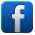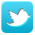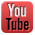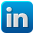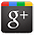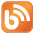Contact us Support: https://support.8x8.com